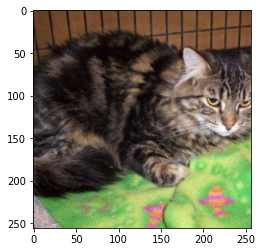### How to Normalize Image Dataset in PyTorch

PyTorch provides a very useful package called "torchvision" for data preprocessing. The colored images have pixel values between 0 and 255 for all three channels. Image transformation is a process to change the original values of the image pixels to a set of new values. The normalization of an image dataset is a very good practice when we work with deep neural networks. Normalizing the image dataset means transforming the images into such values that the mean and standard deviation of the image dataset become 0.0 and 1.0 respectively. To do this first the channel mean is subtracted from each input channel and then the result is divided by the channel standard deviation

output[channel] = (input[channel] - mean[channel]) / std[channel]

In PyTorch, normalization is  done using torchvision.transforms.Normalize() transform. This transform normalizes the tensor images with mean and standard deviation.

Steps for Normalizing Image Dataset in PyTorch:

## Load images/ dataset without normalization

To load a custom image dataset, use torchvision.datasets.ImageFolder()

The images are arranged in the following way:

```root/class_1/xxx.png
root/class_1/xxy.png
root/class_1/[...]/xxz.png

example:
dataset/cat/101.png
dataset/cat/1002.png
dataset/cat/[...]/1000.png```
Use the following Python3 program to load the image dataset.
`import torchvisionfrom torchvision import transformsfrom torch.utils.data import DataLoaderdata_path = './dataset/'transform_img = transforms.Compose([    transforms.Resize(256),    transforms.CenterCrop(256),    transforms.ToTensor(),    # here do not use transforms.Normalize(mean, std)])image_data = torchvision.datasets.ImageFolder(  root=data_path, transform=transform_img)image_data_loader = DataLoader(  image_data,   batch_size=len(image_data),   shuffle=False,   num_workers=0)`

We loaded the image dataset without normalizing the images and we got a dataloader.

#### Visualize an image

We visualize an image from the image dataset.

`# Python code to visualize an imageimport matplotlib.pyplot as pltimages, labels = next(iter(image_data_loader))def display_image(images):  images_np = images.numpy()  img_plt = images_np.transpose(0,2,3,1)  # display 5th image from dataset  plt.imshow(img_plt)display_image(images)`

Output:Image before Normalization

## Calculate the mean and standard deviation of the dataset

When the dataset is small and the batch size is the whole dataset. Below is an easy way to calculate when we equate batch size to the whole dataset.

`# python code calculate mean and stdfrom torch.utils.data import DataLoaderimage_data_loader = DataLoader(    image_data,    # batch size is whole dataset    batch_size=len(image_data),    shuffle=False,    num_workers=0)def mean_std(loader):  images, lebels = next(iter(loader))  # shape of images = [b,c,w,h]  mean, std = images.mean([0,2,3]), images.std([0,2,3])  return mean, stdmean, std = mean_std(image_data_loader)print("mean and std: \n", mean, std)`

Output

`mean and std:(tensor([0.5125, 0.4667, 0.4110]), tensor([0.2621, 0.2501, 0.2453]))`

If our dataset is large and we divide the dataset into batches we can use the below python code to determine the mean and standard deviation.
`# python code to calculate mean and std import torchfrom torch.utils.data import DataLoaderbatch_size = 2loader = DataLoader(  image_data,   batch_size = batch_size,   num_workers=1)def batch_mean_and_sd(loader):        cnt = 0    fst_moment = torch.empty(3)    snd_moment = torch.empty(3)    for images, _ in loader:        b, c, h, w = images.shape        nb_pixels = b * h * w        sum_ = torch.sum(images, dim=[0, 2, 3])        sum_of_square = torch.sum(images ** 2,                                  dim=[0, 2, 3])        fst_moment = (cnt * fst_moment + sum_) / (                      cnt + nb_pixels)        snd_moment = (cnt * snd_moment + sum_of_square) / (                            cnt + nb_pixels)        cnt += nb_pixels    mean, std = fst_moment, torch.sqrt(      snd_moment - fst_moment ** 2)            return mean,std  mean, std = batch_mean_and_sd(loader)print("mean and std: \n", mean, std)`

Output

`mean and std:(tensor([0.5125, 0.4667, 0.4110]), tensor([0.2621, 0.2501, 0.2453]))`

## Normalize the image dataset

To normalize the image dataset we use the above calculated mean and std.

(tensor([0.5125, 0.4667, 0.4110]),

tensor([0.2621, 0.2501, 0.2453]))

If our dataset is more similar to ImageNet dataset, we can use ImageNet mean and std. ImageNet mean and std are mean=[0.485, 0.456, 0.406], std=[0.229, 0.224, 0.225]. If the dataset is not similar to ImageNet like medical images, then calculate the mean and std of the dataset and use them to normalize the images. But it is always advisable to calculate custom mean and std for any type of dataset.

`# python code to normalize the imageimport torchvisionfrom torchvision import transformsfrom torch.utils.data import DataLoaderdata_path = '/dataset/'transform_img_normal = transforms.Compose([    transforms.Resize(256),    transforms.CenterCrop(256),    transforms.ToTensor(),    transforms.Normalize(mean = [0.5125,0.4667,0.4110],                         std= [0.2621,0.2501,0.2453])])image_data_normal = torchvision.datasets.ImageFolder(  root=data_path,   transform=transform_img_normal)image_data_loader_normal = DataLoader(  image_data,   batch_size=len(image_data),   shuffle=False,   num_workers=0)`
We have normalized the images with mean and std calculated above. We get a data loader for the normalized dataset.

Now visualize the normalized image.

`images_normal, labels = next(iter(image_data_loader_normal))display_image(images_normal)`

## Again Calculate the mean and std for the normalized dataset

We calculate the mean and std again for normalized images/ dataset. Now after normalization, the mean should be 0.0, and std be 1.0.

`mean_normal, std_normal = mean_sd(image_data_loader_normal)print("mean and std after normalize:\n",      mean_normal, std_normal)`

Output:

`mean and std after normalize: (tensor([-2.0086e-07, 1.0182e-07, -1.4073e-07]), tensor([1.0000, 1.0000, 1.0000]))`

Here we find that after normalization the mean is 0.0 and the standard deviation is 1.0.